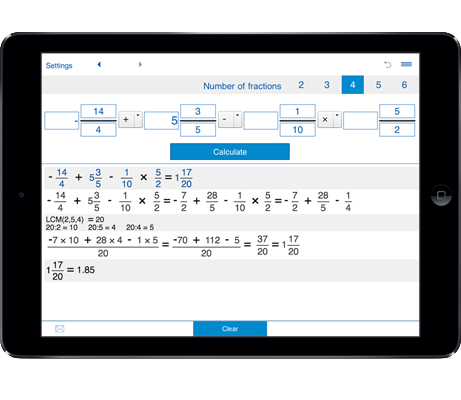# Multiplying fractions calculator

Fractions calculator with steps lets you add, subtract, divide and multiply fractions, mixed fractions, and whole numbers.

## Multiply fractions and mixed fractions, multiply fractions with whole numbers

You can select to multiply 2 fractions, multiply 3 fractions, multiply 4 fractions, multiply 5 fractions, multiply 6 fractions.

### How to use the Multiplying fractions calculator

1. Set the number of fractions you need to multiply - you can select to multiply 2 fractions, 3 fractions, 4 fractions, 5 fractions, or 6 fractions.

2. Enter the fractions, mixed fractions or whole numbers you need to multiply.

3. Click "Calculate".